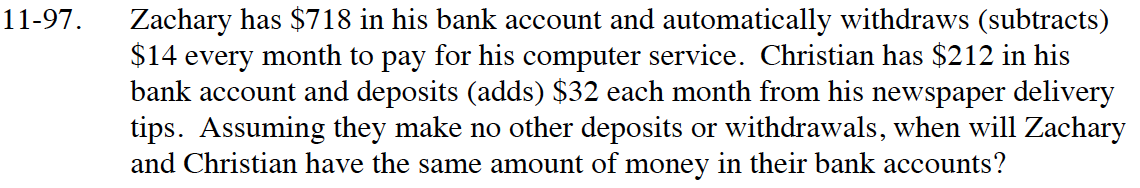### Home > CC4 > Chapter 11 > Lesson 11.2.4 > Problem11-97

11-97.

Zachary has $718 in his bank account and automatically withdraws (subtracts)$14 every month to pay for his computer service. Christian has $212 in his bank account and deposits (adds)$32 each month from his newspaper delivery tips. Assuming they make no other deposits or withdrawals, when will Zachary and Christian have the same amount of money in their bank accounts? Homework Help ✎Create a system of equations that represents the problem.

Define your variables.

Set the equations equal to each other and solve for x.

After 11 months they will have the same balance.

y = total amount of money in the account
x = number of months

y = −14x + 718
y = 32x + 212

−14x + 718 = 32x + 212

506 = 46x

11 = x